# How to Calculate Microscope Magnification?

How to Calculate Microscope Magnification?In this article, let's take a look at how small an object can be seen under a microscope? How much magnification can the object you see be magnified?

 Length Unit   First let's remember some of the most commonly used units of length: 1km=1000m, meter 1m=1000mm, millimeter 1mm=1000um, micrometer 1um =1000nm, namometer 1nm =1000pm, picometer

 Common Object Size Then, let's take a look at some common tiny objects, their average size is generally like this:

 Cells Human cells are generally 20-30um in diameter, red blood cells are 6-9um in diameter, and flat cells with a thickness of 1.5-2.5 microns. White blood cells are spherical in diameter of 7-20um. Platelets are discs with a diameter of 1- 4um and a thickness of about 1um, which can be seen under a 400x optical microscope.

 Bacteria Most bacteria are between 0.5 and 5 μm in diameter, and can be seen with an optical microscope at 1000x magnification

 Virus The diameter of the virus is mostly between 10-300nm, which is difficult to be observed by ordinary light microscope, and part of the appearance can be observed by dark field microscope. Requires an electron microscope to observe.

 Atom Atom diameter is less than 0.1nm, only visible with electron microscope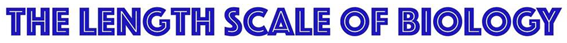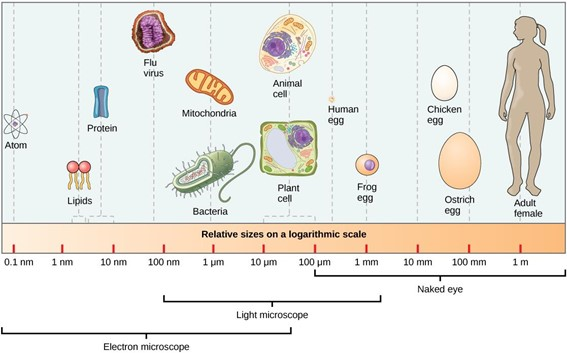Common Resolutions Why can the human eye, optical microscope, and electron microscope observe objects of different sizes? It differs depending on their limiting Resolution:

 Human Eye The resolution of the Human Eye is 0.2mm=200um, the minimum resolution is 0.073mm=73um

 Optical Microscope The resolution of the Optical Microscope is 0.4~0.2um micrometer = 400~200nm nanometer. These are divided into several categories: • Bright Field Microscope resolution 0.4um, • Fluorescence Microscope Ultraviolet Band Resolution 0.2um • Dark Field Microscope can see extremely small objects of 0.02-0.004um.

 Electron Microscope Electron Microscope Resolution can reach 0.0045um~0.001um, say 4.5nm~1nm. (eg A63.7062 4.5nm, A63.7081 1nm)

 What Is Resolution? Resolution refers to the ability to distinguish the minimum distance between two adjacent object points, which is called the resolution distance. Generally speaking, the smaller the resolution distance, the higher the resolution. General regulations: The ability of a microscope or human eye to clearly distinguish the smallest interval of the fine structure of the object under inspection at a photopic distance of 25cm is called resolution. The problem of the resolution limit of optical microscopes was solved by German physicist Abbe in 1873. The eyepieces and objective lenses used in optical microscopes are actually convex lenses. Airy disks are generated when light passes through the convex lenses. A point we see through the microscope is actually a light spot. If the two points to be observed are far apart, we can still tell the difference. But if these two points are very, very close, so close that the two Airy disks they produce overlap, then we can't tell whether they are two points, and we can only see blur of a group. So the size of the Airy disk actually determines the resolution limit of the microscope. The formula for the resolution of an optical microscope is as follows: δ=0.61λ/(nSinα) δ：resolution λ：wave length n：refractive index α：aperture angle After simple conversion, this formula is approximately equal to 1/2 λ, which means that one-half of the wavelength is actually the resolution limit of an optical microscope. Later generations defined it as the "Abbe limit". The shortest wavelength in visible light -- violet light, is about 400 nm, so the Abbe limit is about 200 nm. That is to say, if the distance between two points is less than 200 nanometers, the two points cannot be distinguished by an optical microscope, which is the resolution limit of an optical microscope.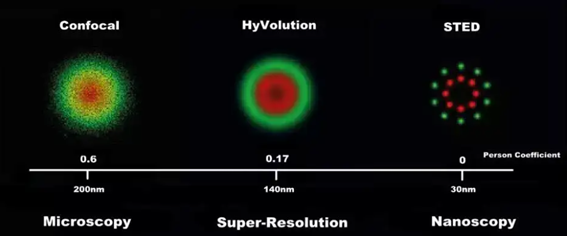Magnification Calculation The formula for calculating the magnification of a microscope is very simple: Total Magnification = Optical Magnification x Digital Magnification

 Optical Magnification Optical magnification refers to the product of the magnification of all optical elements involved in optical imaging in the optical path of the microscope. Generally, it includes eyepiece, objective lens, C-mount, intermediate zoom body, additional objective lens, additional eyepiece, etc. Calculated as follows: Optical Magnification = Eyepiece x Objective x C Mount x Media Lens For example, microscope A33.5121, eyepiece 10x, objective 4x, 10x, 40x, 100x, trinocular C-Mount 0.3x, 1. Optical Magnification observed through binocular eyepiece, 10x (4x~100x)=40x~1000x. 2. Optical Magnification observed through the trinocular tube, 0.3x (4x~100x) =1.2x~30x.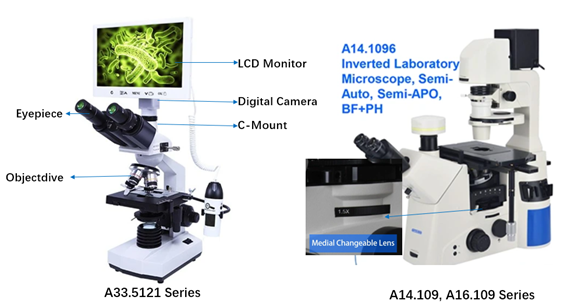Digital Magnification Digital magnification refers to the magnification obtained after the image captured by the imaging chip of the digital camera sensor is outputed to the LCD display. The calculation formula is as follows: Digital Magnification = LCD Diagonal Size / Digital Camera Sensor Diagonal Size For example, digital microscope A33.5121, the diagonal size of digital camera 1/2.75" CMOS chip is 16mm x 1/2.75"=5.81mm, the diagonal size of 7" LCD screen is 25.4mm x 7"=177.8mm, So the digital magnification is 177.8mm/5.81mm=30.6x. Then we can caculate the total magnfication of A33.5121 as: Optical (1.2x~30x) x Digital 30.6x=Total 36.72x~918x

 Attention: The calculation of the diagonal length of CMOS chips is a bit special, because the common specification in the camera industry is 1-inch CCD size = length 12.8mm × width 9.6mm = the corresponding area of the diagonal is 16mm, so when the chip SENSOR calculates the size , not according to the simple length conversion unit 1 inch = 25.4mm, but calculated according to 1 inch = 16mm. You can refer to the following table to quickly find the diagonal lengths corresponding to different chip sizes.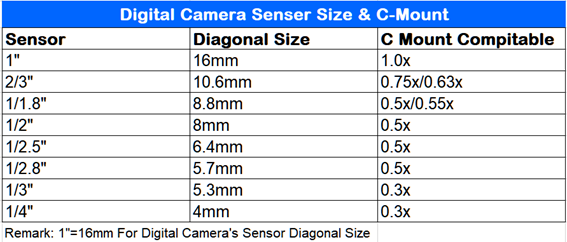Field of View Diameter In addition, in order to make the image captured by the digital camera as close as possible to the field of view observed by the microscope eyepiece, it is necessary to select C-mounts with different magnifications according to the size of the digital camera sensor. The above table also lists digital cameras with different chip sizes, which need to be configured with C-Mount of different magnifications. Field of View Diameter = Eyepiece Field of View / Magnification In the optical microscope, if the configured eyepiece is WF10x/22mm, that is, the number of fields of view is 22mm, and the actual field of view diameter when using a 40x objective lens is 22mm/40x=0.55mm In the digital microscope, if the appropriate C-Mount is selected, and the entire field of view of the CCD sensor can be seen, the calculation method should be similar. For example, the size of CCD chip is 8mm, with 0.5x CMount, 100x objective lens, the actual field of view is 8mm/0.5x/100x=0.16mm Example of Magnification Calculation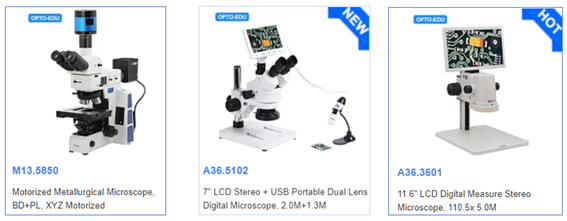M13.5850 Eyepiece PL10x/25mm, Objective BD DIC Semi-APO 5x10x20x50x100x, C-Mount 0.5x, Digital Camera 20M, 1"CMOS，If equipped with 24" LCD monitor Eyepiece observation total magnification =10 x (5x~100x)=50x~1000x Trinocular digital total magnification =0.5x (5x~100x) x (24"x25.4mm/1"x16mm)=95.25x~1905x A36.5102 Eyepiece WF10x, Objective 0.7x~4.5x, C-Mount 0.35x, Digital Camera 2.0M, Eyepiece observation total magnification =10 x (0.7x~4.5x)=7x~45x Trinocular digital total magnification =0.35x (0.7x~4.5x) x (7"x25.4mm / 1/3"x16mm)=8.17x~52.5x A36.3601 No eyepiece, Zoom Lens0.7x~4.5x, Built-in 0.45x Zoom Lens, Digital Camera 5.0M 1/3" CMOS, 11.6" LCD LCD total magnification=0.45x (0.7x~4.5x) x (11.6"x25.4mm / 1/3"x16mm)=17.2x~110.5x

## Service## WeChatWelcome WeChat!

Empty List

Empty List